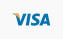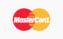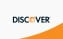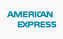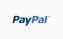Blog Home How Long Will It Take To Heat My Pool?

# How Long Will It Take To Heat My Pool?

by
Categories: How To Guides, Q&A Topics, Heaters

How long will it take to heat my pool? This is a question many people want to know, it is both simple and complex as there are variables to consider. The simple answer is to plug and play with this basic formula:

Gallons of water (GW) multiplied by the weight per gallon (8.33lbs) multiplied by the degrees desired divided by the BTU output of the heater.

For example, a 20,000 gallon pool heated 20 degrees with a heater output of 336,000 BTUs would look like the following:

20,000 x 8.33 = 166,600 lbs of water
166,600 x 20 = 3,332,000 BTUs needed to heat the water 20 degrees
3,332,000 ÷ 366,000 = 9.91 hours

The variables include factors like ambient air and water temperature, wind speed, hours of sun, if it is direct or in the shade, surface area exposed to the elements and where you are located. Needless to say, if you are located at the north pole and its zero degrees outside, the pool is 40 degrees in the middle of winter and there is a 10 mile an hour wind it’s going to take a lot longer to heat your swimming pool than it would if you are at the equator in the middle of summer.

This is another question frequently asked and again there are variables to plug and play. The constant in the equation is the therms per hour. There are 100,000 BTUs per hour for every one therm. Most gas companies use therms as a common measurement of gas used. So, a 400,000 BTU heater will use four therms per hour of operation. The equation would be the number of therms multiplied by the cost of your gas. If your gas costs you \$1.20 per therm and we used the heating time from above at 9.91 hours we would get the following:

4 x \$1.20 = \$4.80
\$4.80 x 9.91 hours = \$47.57 is what it would cost you to heat the water initially

The maintenance cost would be less as you are maintaining that heated water and the variables listed above can play an enormous factor in keeping your pool warm.

But, But... I use propane and not natural gas!
Ok, so the equation is altered a bit because propane is calculated into cubic feet instead of therms. One cubic foot of propane is equivalent to 92000 BTUs. A 400,000 BTU heater will use 4.34 gallons of propane per hour.

400,000 ÷ 92,000 = 4.34
Lets say the propane costs you \$3.00 per gallon, so 4.34 x \$3.00 = \$13.02 per hour
\$13.02 x 9.91 hours = \$129.00 is what it would cost you to heat using propane

As mentioned, the variables can play a big factor in the equations. Many of these examples are based on a 70 degree day with full sun and a wind speed of 3.5 mph. Kick up the wind speed to 11 mph and the energy use goes up 25%. Your location, time of year, elevation above sea level, use of a solar pool cover, surface area exposed to the elements, the depth of the pool and whether it is an above ground or inground pool are all considerations. The type of gas used, the price per BTU and the age of your heater add to the complexities.

So, how fast and how much does it cost to heat your pool? What a great question!

If you need help with this question or others, give us a call, we're here to help!